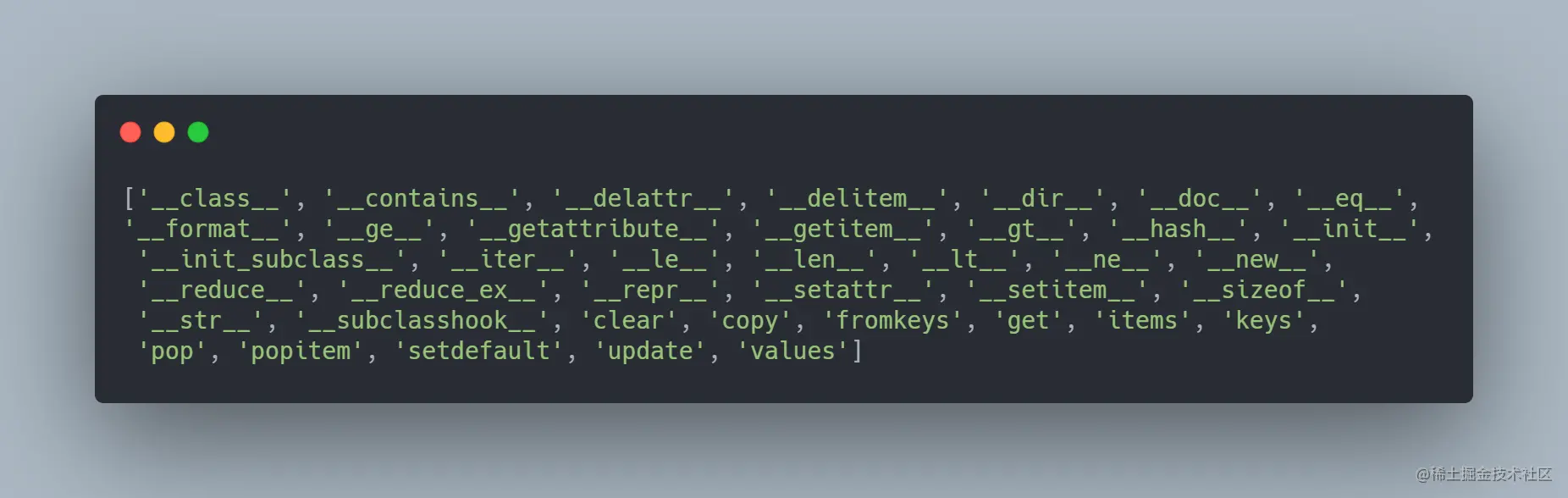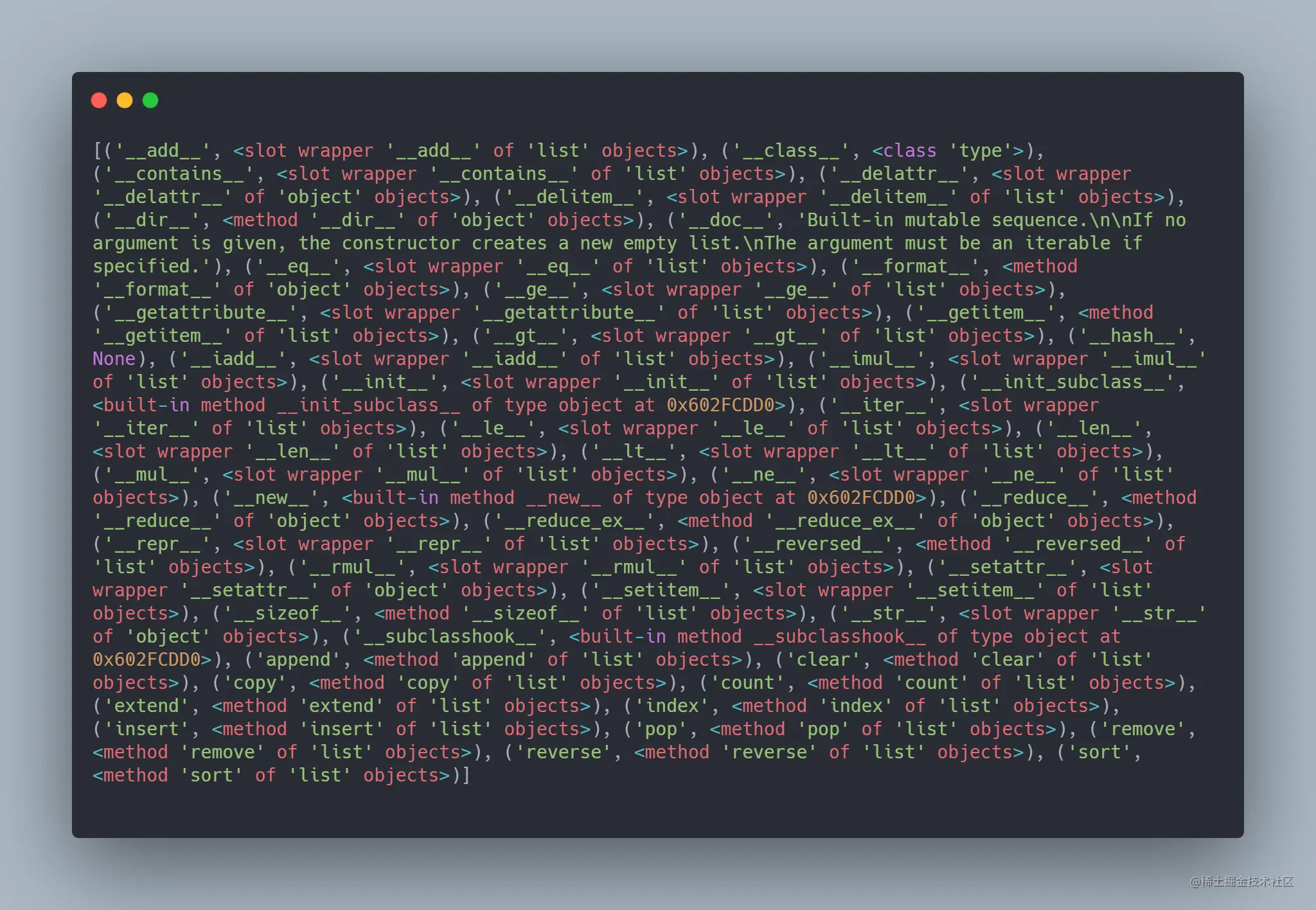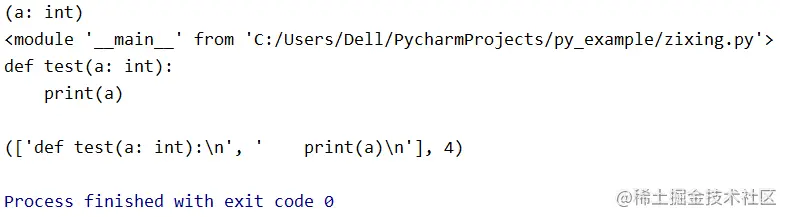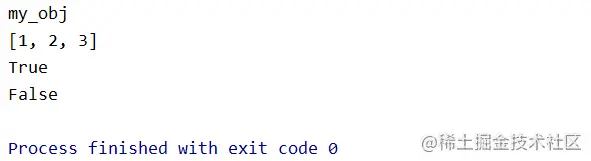## dir()函数

``user = {"nickname": "tigeriaf", "level": 2}print(dir(user))``## type()函数和id()函数

type()函数返回一个对象的类型。例如：

``print(type('tigeriaf'))# 结果输出为 <class 'str'>print(type(2))# 结果输出为 <class 'int'>print(type([1, 2, 3]))# 结果输出为 <class 'list'>``

id()函数返回对象的唯一标识符，是一个整数，在CPython中id()函数用于获取对象的内存地址。例如：

``print(id('tigeriaf')) # 结果输出为 51064768``

## inspect模块

inspect是Python的标准库，提供了更加强大的自省能力，提供了很多函数帮助获取对象的信息，例如模块、类、方法、函数、回溯、帧对象以及代码对象。

### getmembers(object, predicate=None)函数

``import inspect print(inspect.getmembers(list))``### getsourcelines(object)函数

``import inspect  def test(a: int):    print(a)  print(inspect.signature(test))print(inspect.getmodule(test))print(inspect.getsource(test))print(inspect.getsourcelines(test))``## hasattr()函数和getattr()函数

dir()函数会返回某个对象所有属性的列表，但是如果只想测试一个或多个属性是否存在，就需要hasattr()函数和 getattr()函数来完成了，它们的功能分别为判断对象是否有某个属性、获得某个属性值。 例如：

``class MyObj:    def __init__(self):        self.name = 'my_obj'        self.a = 1        self.b = [1, 2, 3]  myobj = MyObj()print(getattr(myobj, "name"))print(getattr(myobj, "b"))print(hasattr(myobj, "a"))print(hasattr(myobj, "c"))``